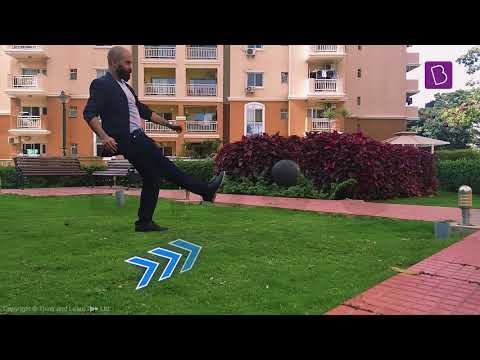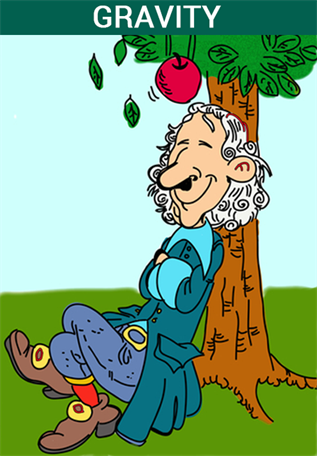# Effects of Forces

## Introduction:

A force is a push or pulls that an object experiences due to its reaction with another object. Force is a vector quantity which affects the motion of an object. There are a variety of forces acting in the universe. The effects of forces are felt by everything and everyone.You may also want to check out these topics given below!

## Types of Forces and their Effects

Broadly speaking, forces can be categorized into two categories:

• Contact force
• Action-at-a-distance force

These two categories of forces affect objects differently.

## What is Contact Force?

A contact force is any force that requires contact to occur. If two surfaces are not in contact, they can’t exert a normal force on each other. Some types of contact forces are listed below.

### Applied Force:

It is the direct force applied to a body by another body.

Example: When you push a bottle across a table, it is an example of applied force.

### Frictional Force:

Friction is the force experienced by an object when it moves or tries to move on a surface. It is a resisting force.

Example: Resistance experienced by a kid while coming down a slide or by a ball rolling on concrete.

### Air Resistance Force:

Air resistance is a type of frictional force experienced by objects moving through air. Like normal friction, air resistance is also resistive by nature. The power of this force depends on the speed of the object as well as its surface area.

Example: A skydiver using a parachute experiences air resistance which slows him down and allows him to land safely.

### Tension Force:

Tension is the force experienced by a fully stretched object such as a string. Tension force acts along the wire and exerts equal pressure at each end.Example: The tension in the string of a guitar decides the nature of its vibration and as a result, the nature of the sound it will make.

### Spring Force:

It is the force exerted by a spring when it is compressed or stretched.

Example: If you stretch a spring and then leave it, it will return to its original position as a result of spring force.

## What is Action-at-a-distance Force?

Action at a distance is the concept that an object can be moved without being physically touched by another object. Some types of action-at-a-distance forces are listed below.

### Gravitational Force:

It is an attractive force exerted by large bodies such as planets and stars.

Example: The solar system is a result of the Sun’s gravitational force on the planets.### Magnetic Force:

It is the force which a magnetic object exerts on ferromagnetic materials or other magnets.

Example: The door of a fridge remains shut due to the magnetic force between the door and body of the fridge.

### Electrical Force:

It is the force experienced by all electrically charged particles in the universe. It can be both attractive and repulsive in nature based on the charge of the particles.

Example: The standing up of hair due to cold or any other sensation is an example of electrical force.

Test your knowledge on Effect of force